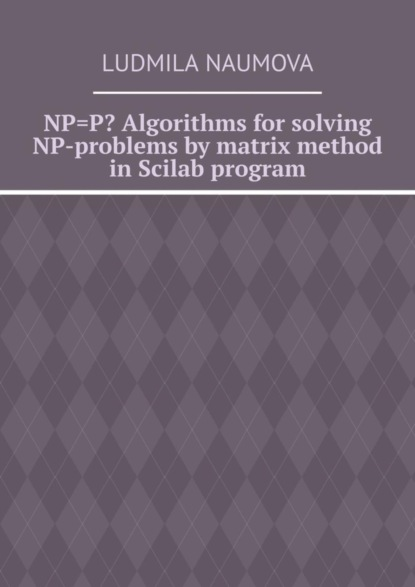﻿ NP=P? Algorithms for solving NP-problems by matrix method in Scilab program (Ludmila Naumova) – скачать в fb2, epub, pdf на FenzinСкачать полностью

# NP=P? Algorithms for solving NP-problems by matrix method in Scilab program

Электронная книга
Добавлено 04.05.2020
Год издания: 2018
Оценка: 0.0
Рецензии в лоцмане: нет рецензий
Скачать: pdf, a6.pdf, epub, fb2, fb3, rtf.zip, txt, txt.zip
Аннотация

We know the problems of combinatorics, such as the problem of permutations, combinations, placement, represented by the corresponding formulas. But these formulas only give us the number of solutions, not the solutions themselves. There were no common standard algorithms for solving these types of problems. These types of problems with large numbers can be referred to NP problems. But with the help of Scilab program typical algorithms of such problems are revealed and solutions are given.

Полная версия

## Читать онлайн

﻿
Отзывы о книге NP=P? Algorithms for solving NP-problems by matrix method in Scilab program
Нет отзывов
Добавить отзыв

Чтобы добавить отзыв Вам нужно зарегистрироваться или авторизироваться